# 一元线性回归分析(R语言)

### 计算实例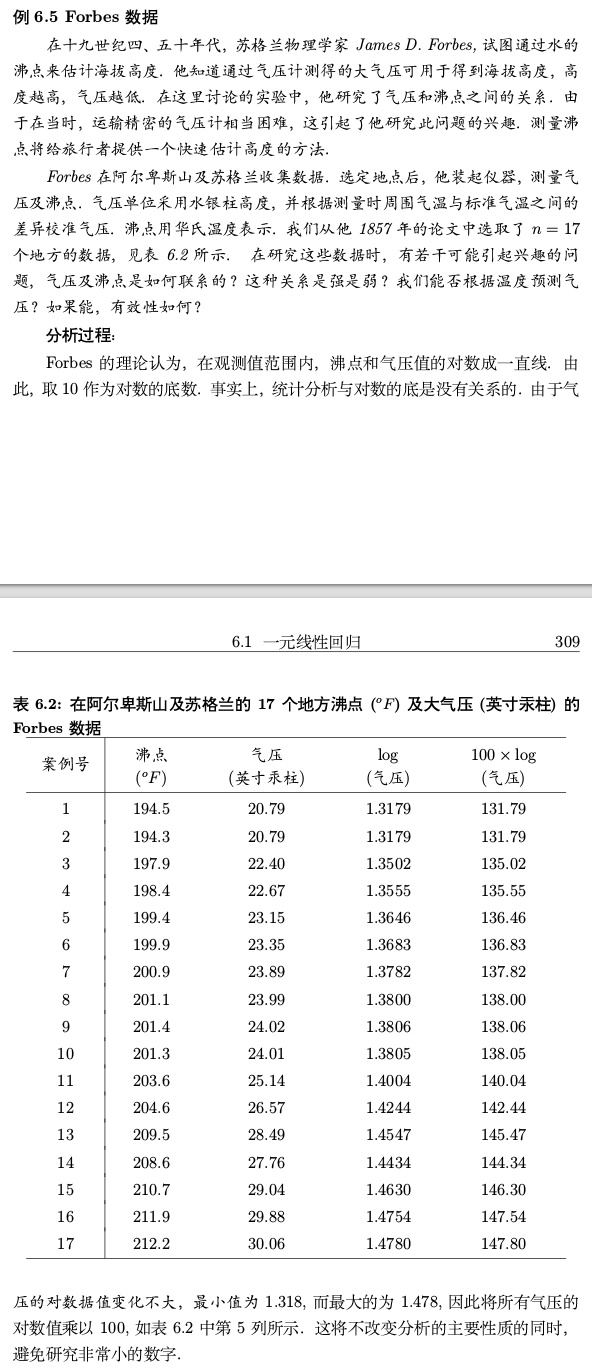#### 画出散点图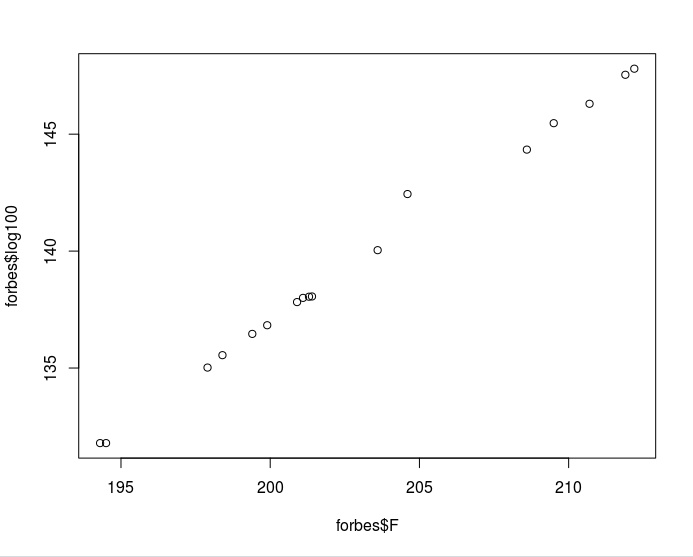$y=ax+b$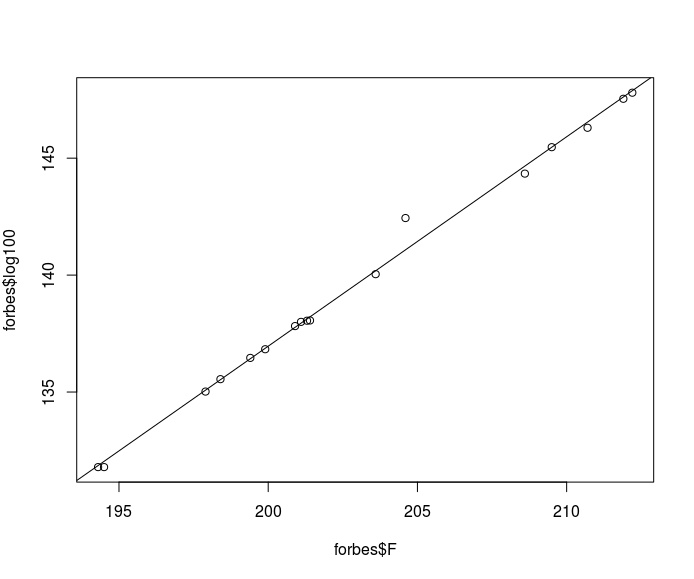#### R是怎么画出这条线的？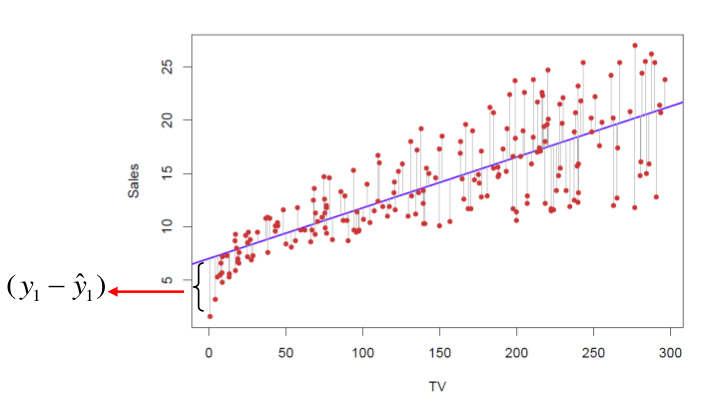$f\left(a,b\right)=\sum_{i=1}^{n}{\left(Y_i - \left(\ aX_i + b \right)\right)^2}$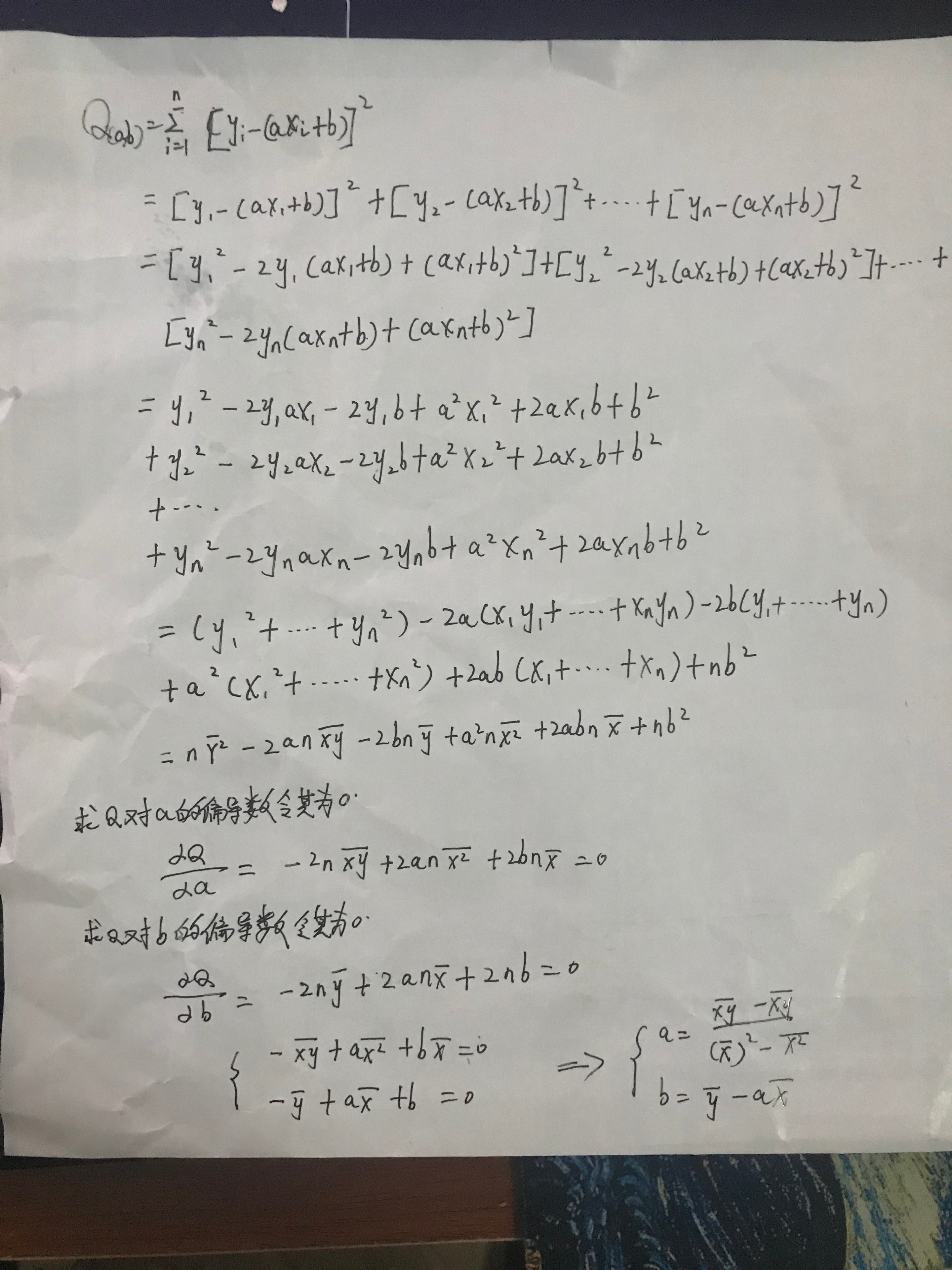$Y=-42.13087 + 0.89546X$

#### 评价回归线拟合程度的好坏

Residuals: 列出了残差的最小值、下四分为数、中位数、上四分为数、最大值

Coefficients : -42.13087表示的是截距、0.89546表示的是斜率,推算的系数的标准差,t值,P-值(结合Signif做显著性检验)

Signif:显著性标记,三颗表示极度显著,二颗表示高度显著,一颗*表示显著,圆点表示不太显著,没有记号表示不显著

Residual standard error : 残差标准查

Multiple R-squared : 相关系数平方

F-statistic: F检验值